# Test: Introduction to Signals

## 10 Questions MCQ Test Topicwise Question Bank for Electrical Engineering | Test: Introduction to Signals

Description
Attempt Test: Introduction to Signals | 10 questions in 30 minutes | Mock test for GATE preparation | Free important questions MCQ to study Topicwise Question Bank for Electrical Engineering for GATE Exam | Download free PDF with solutions
QUESTION: 1

### The signal x(t) = A cos(ωt + φ) is:

Solution:

All bounded periodic signals are power signals, because they do not converge to a finite value so their energy is infinite and their power is finite.

QUESTION: 2

### What is the total energy of the rectangular pulse shown in figure below?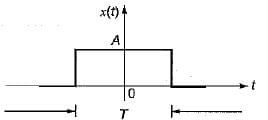Solution:QUESTION: 3

### The auto-correlation function Rx(τ) satisfies which one of the following properties?

Solution:
QUESTION: 4

The auto-correlation function Rx(τ) of the signal x(t) = V sinωt is given by:

Solution: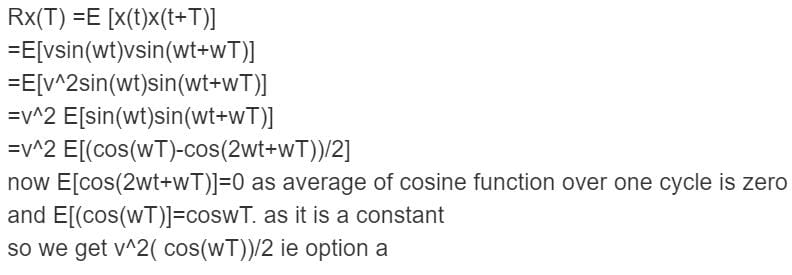QUESTION: 5

The sampling of a function f(t) = sin(2πf0t) starts from zero-crossing. The signal can be detected, if sampling time T is:

Solution:
QUESTION: 6

What is the power and energy of the unit step sequence u(n) respectively?

Solution: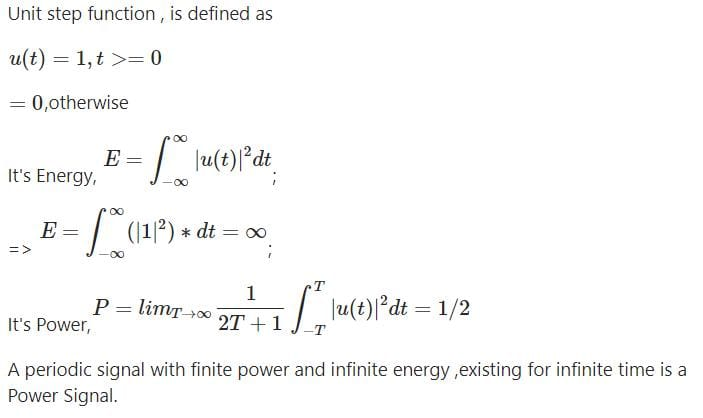QUESTION: 7

Let δ(f) is the delta function the value of integral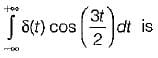Solution:
QUESTION: 8

If a signal f(t) has energy ‘E’ the energy of the signal f(2t) is equal to:

Solution: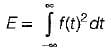and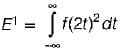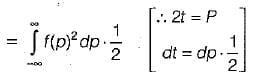⇒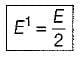QUESTION: 9

Consider the sequence: x[n] = [- 4 - j5, 1 + j2, 4], the conjugate anti-symmetric part of the sequence is:

Solution: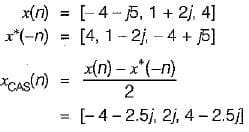QUESTION: 10

The function x(t) is shown in the figure. Even and odd parts of a unit step function u(t) are respectively: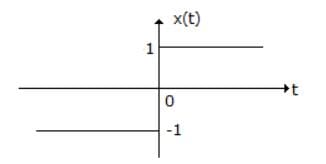Solution: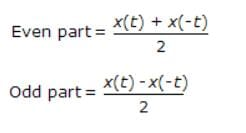Use Code STAYHOME200 and get INR 200 additional OFF Use Coupon Code